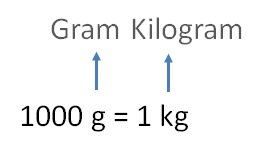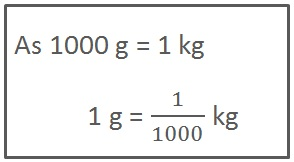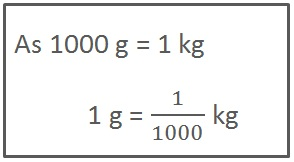### Converting weight - g to kg1 g = 1/1000 kg

### Convert 28 g into kg

28 g  = 28 × 1 g

= 28 × 1/1000 kg= 28/1000 kg

= 0028/1000

= 0.028 kg

### Convert 8560 g into kg

8560 g  = 8560 × 1 g

= 8560 × 1/1000 kg

= 8560/1000 kg

= 8.560 kgSubscribe to our Youtube Channel - https://you.tube/teachoo

1. Chapter 2 Class 7 Fractions and Decimals
2. Concept wise
3. Decimal Numbers

Transcript

Converting weight - g to kg1000 g = 1 kg 1 g = 1/1000 kg Convert 28 g into kg 28 g = 28 1 g = 28 1/1000 kg = 28/1000 kg = 0028/1000 = 0.028 kg Convert 8560 g into kg 8560 g = 8560 1 g = 8560 1/1000 kg = 8560/1000 kg = 8.560 kg

Decimal Numbers